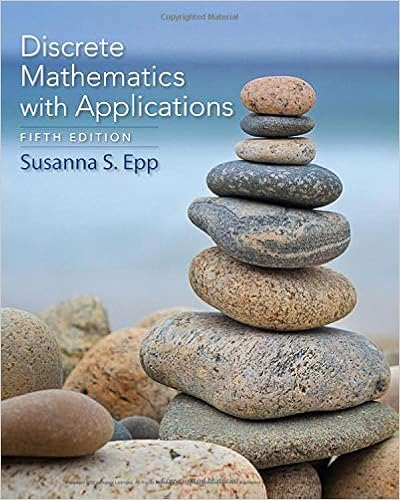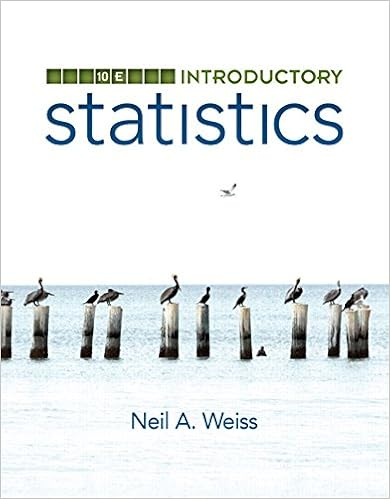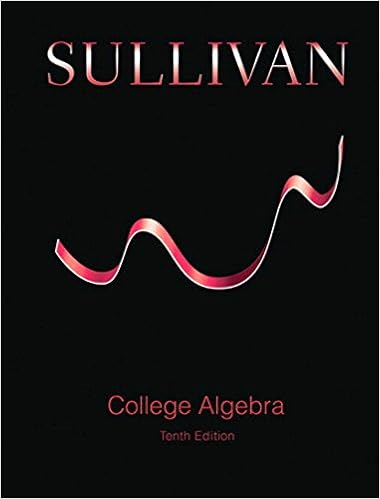# 85164completed - Geometry Writing Assignment: Distance and...

• Homework Help
• 4
• 91% (11) 10 out of 11 people found this document helpful

This preview shows page 1 - 4 out of 4 pages.

GeometryWriting Assignment: Distance and Midpoint FormulasEach problem is worth 5 pointsTotal Points: 50Distance Formula1.Find the distance between the points (-4, 6) and (8, -12).Round your solution to 2 decimal points.2.Find the perimeter of the triangle below. Round your solution to 2 decimal points.
##### We have textbook solutions for you!The document you are viewing contains questions related to this textbook.
Chapter 9 / Exercise 21
Discrete Mathematics With Applications
EppExpert VerifiedBrowse all Textbook Solutions
3.Find the length of diagonal XU in the hexagon below. Round your solution to 2 decimal points.4. Find the distance between the two points. Round your solution to 2 decimal points.
5.How much longer isCDcompared toAB? Round your solution to 2 decimal points.Midpoint Formula6. Find the coordinates of the midpoint ofAB.A (12, -7) and B (-4, 7).7. M is the midpoint ofJK. The coordinates of J are (6, 3) and the coordinates of M are (-3, 4), find thecoordinates of K.
8. Find the midpoint of
End of preview. Want to read all 4 pages?

Course Hero member to access this document

Term
Spring
Professor
N/A
Tags
Distance, decimal points, Elementary geometry, midpoint formulas
##### We have textbook solutions for you!
The document you are viewing contains questions related to this textbook.The document you are viewing contains questions related to this textbook.
Chapter 9 / Exercise 21
Discrete Mathematics With Applications
EppExpert Verified
•••